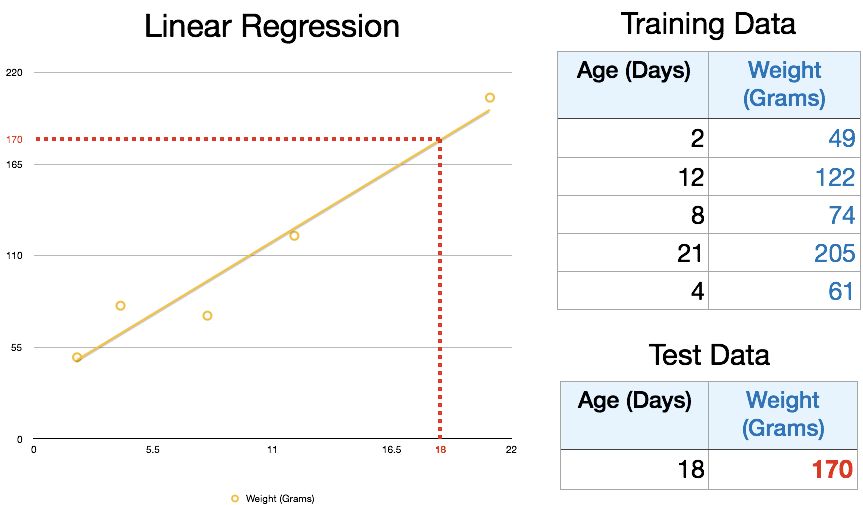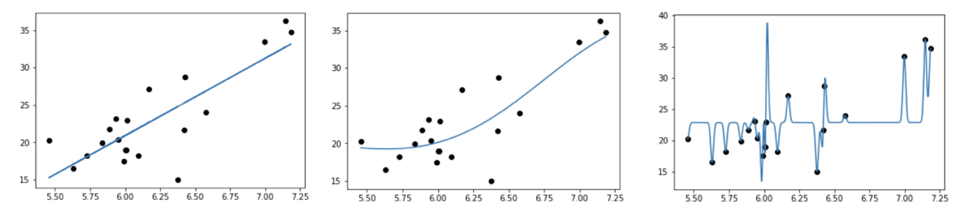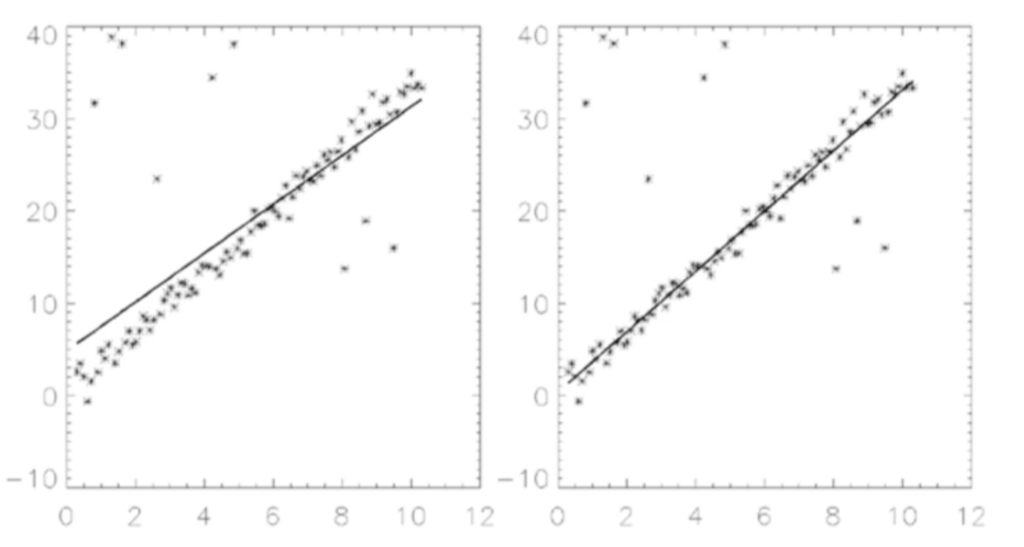## What is Machine Learning?

Introduction to Machine Learning for someone completely new. We don’t get hands on in this lesson but we do get into the details of what machine learning practitioners really care about. Most of the lessons require python, but this lesson has no requirements.

Topic Covered

• The relationship between AI, machine learning, and deep learning
• Linear and multivariate regression
• Training data
• Overfitting
• Loss functions
• The machine learning API

## Statistics

A core part of statistics is linear regression, which is used heavily in machine learning. Training data is given to a model, which is just labelled data points where the input and the correct output are known. The model will then output a number to a new input based on a regression of a line of best fit.We can expand this simple model into a non-linear regression by fitting a more complex equation. However, if we make this model too complex, we see that the line starts to fit the training data very well, but would not be good at predicting new data points. This is known as overfitting. We don’t usually worry too much about overfitting with linear regression because it’s a simple model, but as our equations get more complicated overfitting becomes more and more of an issue. Finding the ideal level of complexity for a problem is a core question of machine learning research.Overfitting: the model on the far left is too simple, but the model on the far right will not generalize to new data

## Loss Functions

An important question in deep learning is what we are optimizing for. Another key concept of machine learning is loss. The loss of a model describes how different our model output is from the output that we wanted. This is used to evaluate the performance of our models – the lower the loss value, the better the model. There are many different ways to calculate error: for instance the diagram on the right shows a model optimized for minimizing absolute error, whereas the diagram on the left is minimizing the square of the absolute error. When you optimize the squared error the outliers affect the line a lot more pulling it away from the majority of the points Whether we use mean squared error (MSE) or absolute error depends on whether we want our model to be more or less affected by outliers.Comparing loss functions: Mean Square Error vs Absolute Error

## The Machine Learning API

The machine learning api is the same as the statistics api, and it is very strict. The input must be a fixed-length list of numbers, and the output must also be a fixed-length list of numbers. Does this mean that we cannot use machine learning on images? Not necessarily, but the input image that is passed to the model must first be converted to a list of pixel values before it can be used. We will learn more about this in the next lecture. Similarly, sound must be processed, often by using its waveform to extract data that we can pass into our model. On text, we use feature extraction to convert text into an array of numbers, by counting the number of times we see each word.Feature extraction converts text into the Machine Learning API

## Choosing an Algorithm

Another question that machine learning engineers grapple with, is what algorithm to use for their particular problem. Linear regression is one algorithm that can be used, but there are many others – such as Naive Bayes, logistic regression, decision trees, neural networks, and many, many more. We will learn about some of these in the upcoming videos, so stay tuned if you’re curious.

So with this knowledge we can now revisit our original question: what counts as a machine learning problem? Anything that can be transformed into the restrictive API of number inputs and number outputs, and has training data. There are many ways we can pre format our data and interpret our output that helps us expand this use case, but if your problem still cannot fit into this definition, then it is probably not suitable for Machine Learning.

In the next tutorial, you will learn to build a Convolutional Neural Network (CNN).